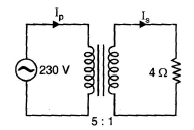Courses

# Test: D.C Machines & Transformers - 1

## 25 Questions MCQ Test Electrical Engineering SSC JE (Technical) | Test: D.C Machines & Transformers - 1

Description
This mock test of Test: D.C Machines & Transformers - 1 for Electrical Engineering (EE) helps you for every Electrical Engineering (EE) entrance exam. This contains 25 Multiple Choice Questions for Electrical Engineering (EE) Test: D.C Machines & Transformers - 1 (mcq) to study with solutions a complete question bank. The solved questions answers in this Test: D.C Machines & Transformers - 1 quiz give you a good mix of easy questions and tough questions. Electrical Engineering (EE) students definitely take this Test: D.C Machines & Transformers - 1 exercise for a better result in the exam. You can find other Test: D.C Machines & Transformers - 1 extra questions, long questions & short questions for Electrical Engineering (EE) on EduRev as well by searching above.
QUESTION: 1

Solution:
QUESTION: 2

Solution:
QUESTION: 3

### A 2000/ 200V, 20 kVA ideal transformer has 66 turns in the secondary. The number of primaryturns is ......

Solution:
QUESTION: 4

The no-load primary current I0 is about ...... of full-load primary current.

Solution:
QUESTION: 5

A transformer takes a current of 0.6 A ad absorbs 64 W when the primary is connected to its normalsupply of 200 V, 50 Hz, the secondary being on open-circuit. The iron loss current is ..........

Solution:
QUESTION: 6

In Figure, the primary will see an impedance of .......Solution:
QUESTION: 7

A 40 kVA transformer has iron loss of 450 W and full-load copper loss of 850 W. If the powerfactor of the load is 0.8 lagging, the full-load efficiency of the transformer is

Solution:
QUESTION: 8

How are transformers generally cooled for installations rated at less than 5 kVA ?

Solution:
QUESTION: 9

The full-load copper losses and iron losses of a transformer are 6400 W and 5000 Wrespectively. The copper loss and iron loss at half full-load will be respectively.

Solution:
QUESTION: 10

The transformer that does not provide electric isolation is

Solution:
QUESTION: 11

What is/ are the advantage/advantages of 3-phase transformer over 3 single-phase bank oftransformers ?

Solution:
QUESTION: 12

The autotransformer is preferably employed in situation where voltage transformetion ratio (K)is

Solution:
QUESTION: 13

The all-day efficiency of a transformer is also called its

Solution:
QUESTION: 14

To produce dynamically induced emf, the following is (are) necessary

Solution:
QUESTION: 15

Function of _____ is to collect current from the commutator and supply it to the external load.

Solution:
QUESTION: 16

A 6 pole wave wound d.c. generator has 460 armature conductors carrying armature current of 180 A. The armature reaction demagnetizing and cross magnetizing ampere turns per pole if brushes are shifted by 6 degree electrical from G.N.A are

Solution:
QUESTION: 17

The motor equation is given by

Solution:
QUESTION: 18

Armature reaction in a d.c. motor is increased......

Solution:
QUESTION: 19

A motor converts

Solution:
QUESTION: 20

Wave winding is employed in a dc machine of

Solution:
QUESTION: 21

The armature MMF waveform of a dc machine is

Solution:
QUESTION: 22

The compensating winding in a dc machine

Solution:
QUESTION: 23

The type of dc generator used for arc welding purposes is a

Solution:
QUESTION: 24

Which type of motors are preferred in lifts?

Solution:

DC compound motors are preferred for lifts. Shunt type commutator motors are preferred in case of single phase installation. The latest lift designs use 3-phase slip ring induction motors with variable frequency drive electronic controls. Some may also use a similar system with permanent magnet (brush less) DC Motors.

QUESTION: 25

What is the increase in torque expressed as percentage of initial torque, if the current drawnby a dc series motor is increased from 10 A to12 A (neglect saturation )?

Solution: